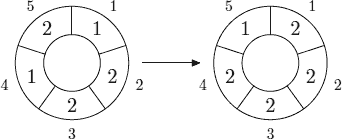Online JudgeProblem SetAuthorsOnline ContestsUser
Web Board
F.A.Qs
Statistical Charts
Problems
Submit Problem
Online Status
Prob.ID:
Register
Authors ranklist
Current Contest
Past Contests
Scheduled Contests
Award Contest
Register
Language:
Cellular Automaton
 Time Limit: 12000MS Memory Limit: 65536K Total Submissions: 4388 Accepted: 1783 Case Time Limit: 2000MS

Description

A cellular automaton is a collection of cells on a grid of specified shape that evolves through a number of discrete time steps according to a set of rules that describe the new state of a cell based on the states of neighboring cells. The order of the cellular automaton is the number of cells it contains. Cells of the automaton of order n are numbered from 1 to n.

The order of the cell is the number of different values it may contain. Usually, values of a cell of order m are considered to be integer numbers from 0 to m − 1.

One of the most fundamental properties of a cellular automaton is the type of grid on which it is computed. In this problem we examine the special kind of cellular automaton — circular cellular automaton of order n with cells of order m. We will denote such kind of cellular automaton as n,m-automaton.

A distance between cells i and j in n,m-automaton is defined as min(|ij|, n − |ij|). A d-environment of a cell is the set of cells at a distance not greater than d.

On each d-step values of all cells are simultaneously replaced by new values. The new value of cell i after d-step is computed as a sum of values of cells belonging to the d-enviroment of the cell i modulo m.

The following picture shows 1-step of the 5,3-automaton.The problem is to calculate the state of the n,m-automaton after k d-steps.

Input

The first line of the input file contains four integer numbers n, m, d, and k (1 ≤ n ≤ 500, 1 ≤ m ≤ 1 000 000, 0 ≤ d < n2 , 1 ≤ k ≤ 10 000 000). The second line contains n integer numbers from 0 to m − 1 — initial values of the automaton’s cells.

Output

Output the values of the n,m-automaton’s cells after k d-steps.

Sample Input

```sample input #1
5 3 1 1
1 2 2 1 2

sample input #2
5 3 1 10
1 2 2 1 2```

Sample Output

```sample output #1
2 2 2 2 1

sample output #2
2 0 0 2 2```

Source

[Submit]   [Go Back]   [Status]   [Discuss]Home PageGo BackTo top